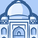# QlikView App Dev

Discussion Board for collaboration related to QlikView App Development.

Announcements
cancel
Showing results for
Did you mean:Contributor III

## 'Other' in dimension limit is grouping all values.

Hi all,

I have an issue where i am taking sum of total working hours of all employees and dividing it with actual working hours per month.

Sum(actual working hours for Jan 2017) = 184, for Feb it's 168 for Mar it's 184.

My expression says : sum(working hours)/ sum(aggr(sum distinct actual_hours),Area,Month)

The 'Other' value is showing wrong, it should be 15 but it's showing 9. Please see the attached qvw.

1 Solution

Accepted SolutionsContributor III
Author

Thanks for the reply Sunny, but my requirement says that i should consider all month irrespective of whether data is present for that month or not. So what i did is i stored this value

sum(aggr(sum(hours),Month)) into a variable and then used this variable in chart expression.

2 RepliesMVP

May be this

Sum(Working_Hours)/If(Len(Trim(Area)) = 0, Sum(Aggr(hours, Month)), Sum(hours))Contributor III
Author

Thanks for the reply Sunny, but my requirement says that i should consider all month irrespective of whether data is present for that month or not. So what i did is i stored this value

sum(aggr(sum(hours),Month)) into a variable and then used this variable in chart expression.